HELSINKI UNIVERSITY OF TECHNOLOGY ABSTRACT OF MASTER'S THESIS
Author: Title of thesis: Kenrick Bingham Mathematics of Local X-Ray Tomography Lokaalin röntgentomografian matematiikkaa 18th August, 1998 Pages: 107 Department of Engineering Physics and Mathematics Mat-1 Mathematics Professor Olavi Nevanlinna Professor Erkki Somersalo This thesis provides a self-contained account of the basic mathematical theory of local x-ray tomography. The theory shows when and how an n-dimensional object (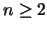) can be reconstructed, using attenuation measurements of x-rays passing through the object. Mathematically speaking, the problem is to determine a compactly supported function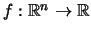from its line integrals. The reconstruction of f itself is a numerically instable operation, but formulae are derived for the stable reconstruction of e * f and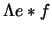, following [SK]. Here e * f andare, under certain assumptions, blurred approximations of f and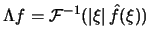, respectively. The Calderón-Zygmund theory needed for deriving the reconstruction formulae is also presented, largely according to [Ner]. The function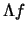provides meaningful information about the internal structure of the object, since the Calderón pseudodifferential operator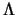preserves the locations of discontinuities. This is shown by proving that the wave front sets of f andare the same. The elementary proof of this fact, which does not use the theory of pseudodifferential operators, is presented as outlined in [RK]. One reason for consideringis that its approximate reconstruction can be done locally. This is to say that if the region of interest is only part of the object examined, measurements are needed only along lines through the region of interest, or very close to it. The functions f and e * f cannot be reconstructed locally. Two stability results not directly given in the references are proved. They state that the errors in the reconstructed e * f andare uniformly bounded by the L2 norm of the error in the measurements. The presentation is self-contained in the sense that only some basic knowledge of real analysis, distribution theory and Fourier analysis is required; some of the most central results of this background theory are listed in an appendix for reference. Apart from these prerequisites, proofs of all results used are given, except for two theorems concerning the continuity of the Riesz transform, for whom the reader is referred to [Zie]. These theorems also imply that the function space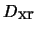, considered in [SK], is just the space of square-integrable functions. Earlier statements of this fact were not found in literature. tomography, computed tomography, CT, local, inverse problems, singular integrals, Calderón-Zygmund theory

## Bibliography

Ner
Umberto Neri.
Singular Integrals.
Number 200 in Lecture Notes in Mathematics. Springer-Verlag, Berlin, Germany, 1971.
ISBN 3-540-05502-9.

RK
A. G. Ramm and A. I. Katsevich.
The radon transform and local tomography.
CRC Press, Boca Raton, U.S.A., 1996.
ISBN 0-8493-9492-9.

SK
Kennan T. Smith and F. Keinert.
Mathematical foundations of computed tomography.
Applied Optics, 24(23):-3957, December 1, 1985.
ISSN 0003-6935.

Zie
William P. Ziemer.
Weakly Differentiable Functions.
Number 120 in Graduate Texts in Mathematics. Springer-Verlag, New York, U.S.A., 1989.
ISBN 0-387-97017-7.

 Kenrick Bingham 12.8.1999